## SUM Function

The SUM function is a premade function in Google Sheets, which adds up numbers in a range.

It is typed `=SUM`and gets a list of cells:

=STDEV.S(value1, [value2, ...])

Note: The `=SUM` function adds up both negative and positive cell values.

Let's see some examples!

## Sum Function Example

Calculate the sum of all the Total stats for this list of Pokemon: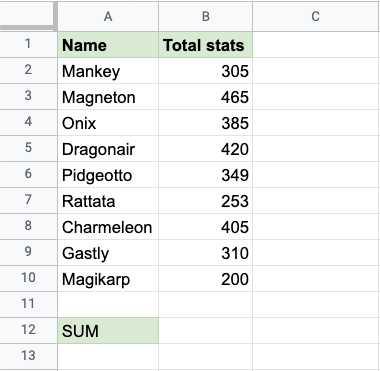Copy Values

The SUMSUM function, step by step:

1. Select the cell `B12`
2. Type `=SUM`
3. Click the SUM command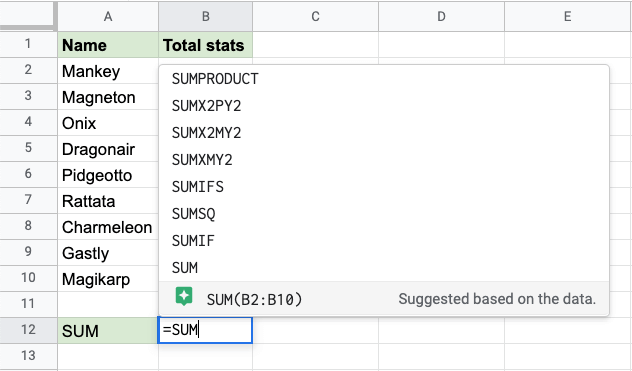1. Specify the range `B2:B10` for the Total stats
2. Hit enter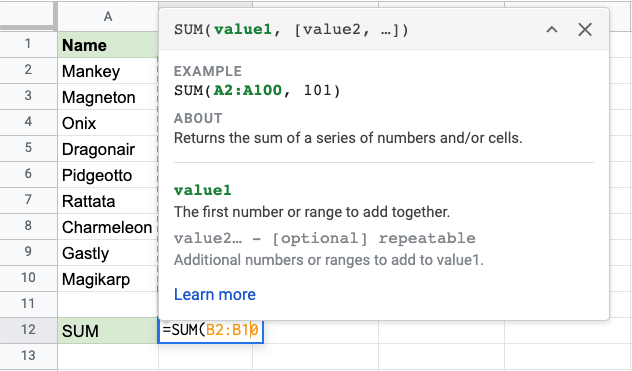Now, the function calculates the sum of Total stats for the Pokemon in the list: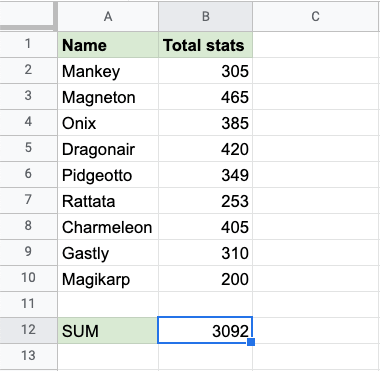Great job! You have successfully calculated the sum of the stats using the `=SUM` function. The Pokemon's stats have a total sum of `3092`.

Let's change one of the values in the range, to see what happens. Type `350` into cell `B7`: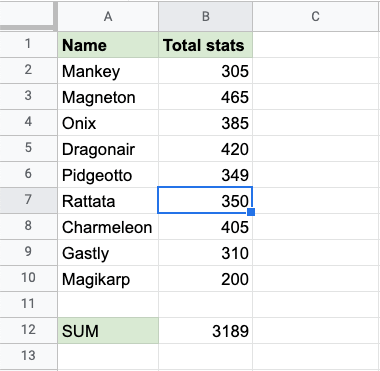Rattata total stats was changed from `253` to `350`.

The SUM function updated the total sum from `3092` to `3189`.

It updates the SUM when values inside of the range are changed.

Lets change Rattata's stats to a negative number to see what happens. Type `-350` into cell `B7`: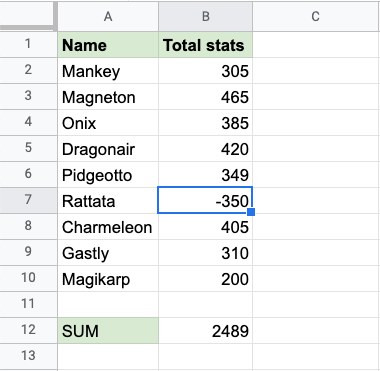The SUM is updated to `2489`. Adding a negative number subtracts that value from the range.

W3Schools is optimized for learning and training. Examples might be simplified to improve reading and learning. Tutorials, references, and examples are constantly reviewed to avoid errors, but we cannot warrant full correctness of all content. While using W3Schools, you agree to have read and accepted our terms of use, cookie and privacy policy.